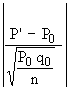Support the Monkey! Tell All your Friends and TeachersHome MonkeyNotes Printable Notes Digital Library Study Guides Study Smart Parents Tips College Planning Test Prep Fun Zone Help / FAQ How to Cite Request a New Title

8.12 Sampling of Attributes

Characteristics like religion, language, locality etc. cannot be measured in numbers as they are attributes. We can only say whether a particular attribute is present or absent in an individual. The sampling of attributes means drawing a sample from the population, of which every member possesses a particular attribute or does not have it. In many cases, we are interested in knowing how many in the population possess the attribute. For instance, Brooke wants to know how many from his area are smokers i.e. he is interested in the number or proportion of smokers in his area. Now we shall see how to test a hypothesis about the number or proportion of the individuals of the population possessing an attribute.

Testing the hypothesis that population proportion p = Po

In notations : Ho : population proportion = Po

Now P' = sample proportion is normally distributed with mean Po and standard deviationHence z =1. If | z | > 1.96 a rare event has taken place.

If P ( | z | > 1.96 ) = 0.05 we reject the hypothesis

= Po at 5% level of significance.

2. If | z | > 1.96 a usual event has happened and accept the hypothesis that P = Po at 5% level of significance.

3. If | z | > 2.58 a rare (unusual) event has taken place. Now P ( | z | > 2.58 ) = 0.01. We then reject the hypothesis P = Po at 1% level of significance.

4. If | z | > 2.58, an unusual event occurred and we accept the hypothesis at 1% level of significance.

Example A sample of 600 persons selected at random from a large city shows that there are 53% smokers. Is there any reason to doubt the hypothesis that smokers and non-smokers are equal in number in the city ?Your browser does not support the IFRAME tag.

Solution: The null hypothesis is that the smokers and non smokers are equal in numbers.

Ho : P = Po and Ha : P ¹ Po
where Po = 0.5 Þ qo = 1 - Po = 0.5

Also the sample proportion is P' = 53% = 0.53 and n = 600.Hence the hypothesis Ho is accepted at 5% level of significance. i.e. we can undoubtedly say that both smokers and non-smokers are equal in numbers in that city.

Index

8.1 Population
8.2 Sample
8.3 Parameters and Statistic
8.4 Sampling Distribution
8.5 Sampling Error
8.6 Central Limit Theorem
8.7 Critical Region
8.8 Testing of Hypothesis
8.9 Errors in Tesitng of Hypothesis
8.10 Power o a Hypothesis Test
8.11 Sampling of Variables
8.12 Sampling of Attributes
8.13 Estimation
8.14 Testing the Difference Between Means
8.15 Test for Difference Between Proportions
8.16 Two Tailed and one Tailed Tests
8.17 Test of Significance for Small Samples
8.18 Students t-distribution
8.19 Distribution of 't' for Comparison of Two Samples Means Independent Samples
8.20 Testing Difference Between Mens of Two Samples Dependent Samples or Matched Paired Observations
8.21 Chi-Square
8.22 Sampling Theory of Correlation
8.23 Sampling Theory of Regression

Chapter 1Search: All Products Books Popular Music Classical Music Video DVD Toys & Games Electronics Software Tools & Hardware Outdoor Living Kitchen & Housewares Camera & Photo Cell Phones Keywords: